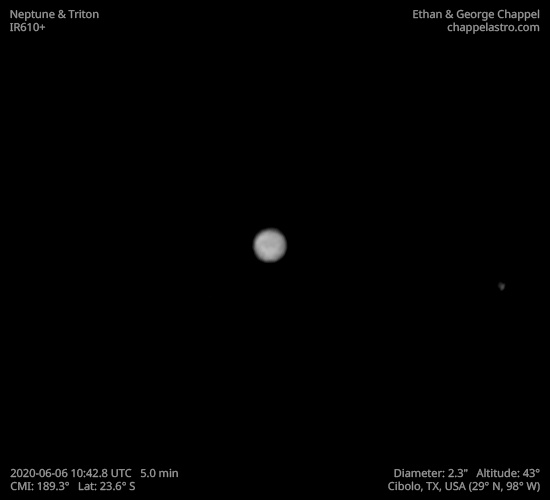# Neptune 2020-06-06 10:42 UTC

CM1: 189.30°

CLat: -23.60°

Description

First time going after Neptune with the C14 and first light with a Baader Red filter, which allows more infrared light through than the 685nm filter. I cut my time with the ice giant short to have enough time for Mars.

Notes:

• Much of the southern hemisphere may be brighter than the rest of the planet.

Logs
```FireCapture v2.6  Settings
------------------------------------
Observer=Ethan Chappel
Location=Cibolo
Scope=Celestron C14 EdgeHD
Camera=ZWO ASI290MM
Filter=L
Profile=Neptune
Diameter=2.23"
Magnitude=7.90
FocalLength=7750mm (F/21)
Resolution=0.08"
Filename=2020-06-06-1042_8-EC-L-Neptune.ser
Date=060620
Start=104020.307
Mid=104250.331
End=104520.356
Start(UT)=104020.307
Mid(UT)=104250.331
End(UT)=104520.356
Duration=300.049s
Date_format=ddMMyy
Time_format=HHmmss
LT=UT -6h
Frames captured=3994
File type=SER
Binning=no
ROI=1024x768
ROI(Offset)=0x0
FPS (avg.)=13
Shutter=75.00ms
Gain=550 (91%)
HighSpeed=off
AutoGain=off
USBTraffic=100 (off)
SoftwareGain=10 (off)
FPS=100 (off)
Brightness=1 (off)
Gamma=50
AutoExposure=off
AutoHisto=75 (off)
Histogramm(min)=0
Histogramm(max)=110
Histogramm=43%
Noise(avg.deviation)=2.96
Limit=300 Seconds
Sensor temperature=31.2°C
Focuser position=860
```